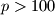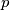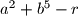### IMO Shortlist 2012 problem N8

Kvaliteta:
Avg: 0.0
Težina:
Avg: 9.0
Prove that for every prime$p>100$ and every integer$r$, there exist two integers$a$ and$b$ such that$p$ divides$a^2+b^5-r$.
Source: Međunarodna matematička olimpijada, shortlist 2012